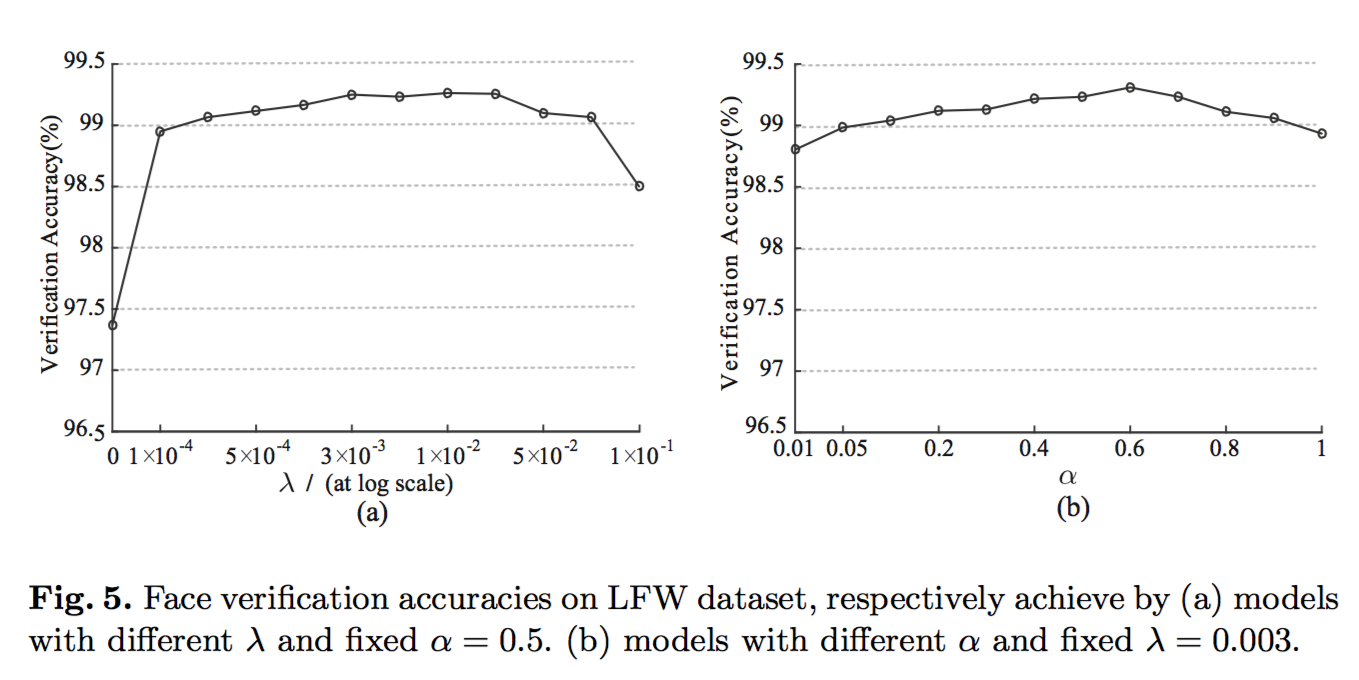## Contrastive Loss

Contrastive Loss（对比损失）我第一次接触是在 Caffe 的 Siamese Network（孪生神经网络），当时对这个网络一知半解，对这个损失函数更是只会调用 API，为此我还写过一篇文档介绍 Siamese Network

Siamese Network 由两个样本组合，可以相同也可以不同，两两配对进行训练。

Contrastive Loss 的公式如下:

$$\mathcal{L}=\frac{1}{2N}\sum_{n=1}^N[yd^2+(1-y)max(margin-d,0)^2]$$

$$d=\sqrt{(v_1-v_2)^2}$$

\mathcal{L}=\left\{\begin{aligned}d^2, && y=1\\max(margin-d,0)^2, && y=0\\\end{aligned}\right.

$margin$ 为预设的阈值，当 $margin-d<0$ 时，$\mathcal{L}=0$。

• 当 $y=1$ 时，$d$ 越大，$\mathcal{L}$ 也就越大。
• 当 $y=0$ 时，$d$ 越小，$\mathcal{L}$ 也就越大。

caffe 中的 contrastive_loss_layer.cpp 实现主要看 Forward_cpuBackward_cpu 两个函数，其他都无关紧要。

for (int i = 0; i < bottom->num(); ++i) {
dist_sq_.mutable_cpu_data()[i] = caffe_cpu_dot(channels,
diff_.cpu_data() + (i*channels), diff_.cpu_data() + (i*channels));
if (static_cast<int>(bottom->cpu_data()[i])) {  // similar pairs
loss += dist_sq_.cpu_data()[i];
} else {  // dissimilar pairs
if (legacy_version) {
loss += std::max(margin - dist_sq_.cpu_data()[i], Dtype(0.0));
} else {
Dtype dist = std::max<Dtype>(margin - sqrt(dist_sq_.cpu_data()[i]),
Dtype(0.0));
loss += dist*dist;
}
}
}


caffe_cpu_dot 对两个向量做内积，具体代码见 math_functions.cpp，参考 梳理caffe代码math_functions(一)cpu_data()返回的是一个指针。

Forward_cpu 计算 $\mathcal{L}$ 和公式一样，区别在于非 legacy_version 使用的是 $margin-d^2$。

caffe 中的 siamese example 将 margin 设为了 1。

## Triplet Loss

Triplet Loss 我在接触 Contrastive Loss 的时候也看过，不过当时看不懂就没有深入，后来吃过很多亏。

Triplet Loss 和 Contrastive Loss 在样本上的差别在于，Triplet Loss 有三个样本配成两对，一对相同和一对不同；Contrastive Loss 两两配对，可以相同也可以不同。

Triplet 翻译成三胞胎，可以把这个 Loss Function 叫做 Triplet Loss，除了训练样本之外，看它的公式:

$$\mathcal{L} = \frac{1}{2N}\sum_i^N[\lVert f(x_i^{anchor})-f(x_i^{pos}) \rVert^2_2 - \lVert f(x_i^{anchor})-f(x_i^{neg}) \rVert^2_2+\alpha]_+$$

Triplet Loss 将一个训练样本分成三部分，一个基准为 anchor，与 anchor 同一类的为 positive，与 anchor 不同的为 negative。

$$\mathcal{L} = RELU(d(x_i^{anchor}, x_i^{pos})-d(x_i^{anchor}, x_i^{neg})+\alpha)$$

RELU(x)=\left \{ \begin{aligned}x, && x>0\\ 0, && x\le 0\\ \end{aligned}\right.

$d(x_i^{anchor}, x_i^{neg})$ 最后肯定要比 $d(x_i^{anchor}, x_i^{pos})$ 要大，$\mathcal{L}$ 不能为 0，所以小于零时取 0。如果直接这样最后的结果必定是 $\mathcal{L}=0$，所以预设一个 $\alpha$ 表明 anchor 和 negative 的距离与 anchor 和 positive 的距离有一个最小的间距。

$\alpha$ 的取值很重要，$\alpha$ 过小时，提取到的特征向量区分不明显，很快就收敛了；$\alpha$ 过大时，很难收敛。

caffe 没有实现 Triplet Loss，但是可以参考 如何在caffe中增加layer以及caffe中triplet loss layer的实现

## Center Loss

So, how to develop an effective loss function to improve the discriminative power of the deeply learned features? Intuitively, minimizing the intra-class variations while keeping the features of different classes separable is the key.

$$\mathcal{L}=\mathcal{L}_S+\lambda \mathcal{L}_C$$

$c_{y_i}$ 代表了 $y_i$ 类数据的特征的中心，当 $x_i$ 改变时 $c_{y_i}$ 也应该改变，因为 $x_i$ 就是 CNN 提取到的特征。

To address this problem, we make two necessary modifications. First, instead of updating the centers with respect to the entire training set, we perform the update based on mini-batch. In each iteration, the centers are computed by averaging the features of the corresponding classes (In this case, some of the centers may not update). Second, to avoid large perturbations caused by few mislabelled samples, we use a scalar α to control the learning rate of the centers.

• 每个 mini-batch 计算 class center，也就是 $c_{y_i}$
• 为了避免非 Ground Truth 的样本影响，引入一个 $\alpha$ 超参控制 center 的学习率

$$\frac{\partial L_C}{\partial x_i}=x_i-c_{y_i}$$

$S$ 为 softmax 函数，$y_j$ 为 N 类的向量，只有一个位置为 1:

$$S=\frac{e^{x_i}}{\sum_{j=1}^N e^{x_j}}$$

$$\frac{\partial L_C}{\partial x_i}=0$$

$$\Delta c_j=\frac{\sum_{i=1}^m\delta(y_i=j)\cdot(c_{y_i}=x_i)}{1+\sum_{i=1}^m\delta(y_i=j)}$$

$\delta(y_i=j)$ 判断条件，如果为真则为 1，否则为 0。

• 计算 $\mathcal{L}=\mathcal{L}_S+\lambda \mathcal{L}_C$

• 计算反向传播的误差，$\frac{\partial L}{\partial x_i}=\frac{\partial L_S}{\partial x_i}+\lambda \frac{\partial L_C}{\partial x_i}$

• 更新 $W$，$W^{t+1}=W^t - \mu^t \frac{\partial L^t}{\partial W^t}=W^t - \mu^t \frac{\partial L_S^t}{\partial W^t}$

• 更新 $c_j$，$c^{t+1}_j=c^t_j-\alpha \Delta c^t_j$

• 更新卷积层参数 $\theta_C$

$$\theta_C^{t+1}=\theta_C^t-\mu^t\sum_i^m\frac{\partial L^t}{\partial x_i^t}\cdot\frac{\partial x_i^t}{\partial \theta_C^t}$$

$\alpha$ 和 $\lambda$ 作者做过很多实验，固定其中之一然后改变另外一个超参: